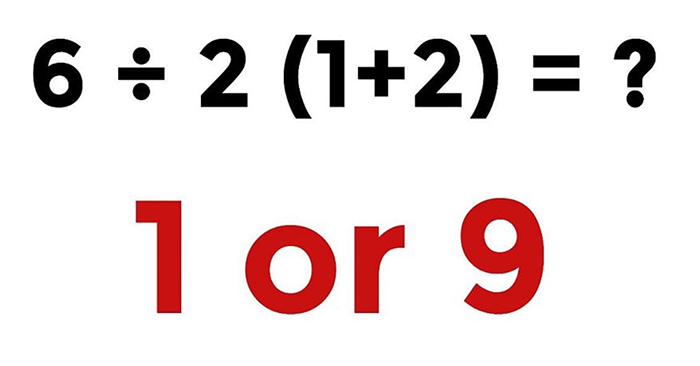Home Lifestyle Are You Smart Enough To Solve This Math Problem?

# Are You Smart Enough To Solve This Math Problem?

Can you figure out the correct solution?

Here is the challenge, in the picture below.

At the top of the picture we see the math problem and then two possible answers.

Which solution do you think is the correct one?How did you come up with it?

Take your time and think about it so you can find the correct solution for the math problem.

Done? Below you can check if you picked the right number!

A

B

CWhy is 9 the correct answer?

6÷2(1+2)

= 6÷2(3)

Well, if you remember from your school days, according to the order of operations, division and multiplication have the same precedence, so the correct order is to evaluate from left to right. First, take 6 and divide it by 2, and then multiply by 3.

6÷2×3

= 3×3

= 9